ISSN ONLINE(2320-9801) PRINT (2320-9798)

All submissions of the EM system will be redirected to Online Manuscript Submission System. Authors are requested to submit articles directly to Online Manuscript Submission System of respective journal.

# Space Vecor Modulated Three Level Neutral Point Clamped Inverter Using A Single Z Source Network

 R.Arjunan1, D.Prakash2 PG-Scholar, Department of Power Electronics and Drives, Sri Ramakrishna Engineering College, Coimbatore, India Assistant Professor, Department of EEE, Sri Ramakrishna Engineering College, Coimbatore, India Related article at Pubmed, Scholar Google

Visit for more related articles at International Journal of Innovative Research in Computer and Communication Engineering

## Abstract

The Neutral Point Clamped (NPC) three-level inverter with Z-source network is a relatively recent converter topology that exhibits both voltage-buck and voltage-boost capability. This concept can be applied to all acto- ac, dc-to-dc, ac-to-dc, and dc-to-ac power conversion whether two-level or multilevel. However, multilevel inverters offer many benefits for higher power applications. Existing publications have shown the control of a Z-source flying capacitor inverter using the carrier-based modulation technique. This paper presents the control of a neutral point clamped three-level inverter with Z-source network using the space vector modulation technique. This gives a number of benefits in both implementation and harmonic performance which enables the operation of the Z-source arrangement to be optimized and implemented digitally without introducing any extra commutations.

### KEYWORDS

Medium Voltage (MV), Neutral point clamped (NPC) inverter , Space vector modulation (SVM), Pulse width modulation (PWM)

### I. INTRODUCTION

With the technology advancements in semiconductor devices such as high-voltage insulated gate bipolar transistors (IGBT) and gate controlled thyristor (GCT), modem multi-megawatt variable frequency drives are increasingly used in petrochemical, mining, steel & metals, transportation and other industries to conserve electric energy, increase productivity and product quality. These are using various power converter technologies in their drive products. For instance, ABB produces three-level neutral point clamped inverter fed drives, Rockwell manufactures current source inverter based drives, Rubicon promotes cascaded H-bridge multilevel inverter technology, Toshiba uses multilevel NPC/H-bridge hybrid inverters, and Alston is developing flying-capacitor multilevel inverter drives. All these drive systems operate at medium voltage (MV) levels, typically from 2300V to 7200V. This thesis focuses on high power three-level neutral point clamped inverter using space vector modulation with even order harmonic elimination. Three level neutral point clamped inverters are used to drive medium voltage high power AC motors because of their high voltage and high power capacity and excellent harmonic performance. Such motor drives are used in many high power variable speed application, such as high power pumps, fans, etc., leading to significant energy savings. In pulse width modulated controlled inverter each inverter leg output voltage is a PWM modulated step wise voltage. 
The preliminary of using multiphase inverters for variable speed drives was proposed with using multiphase machines instead of three phase machines. It has several advantages such as reduction in copper loss, and attenuation of phase belt harmonics. The strategy of generating pulse width modulation switching signals for multi-level multi-phase inverters used in multi-phase machine is an important means of controlling multiphase machines. 
Multi-level inverters are finding increased attention in industries as a choice of electronic power conversion for medium voltage and high power applications, because of improving the output waveform the inverter reduces its respective harmonic content and hence the size of the filter used and the level of the electromagnetic interference generated by switching operation. Many new modulations have been developed to cater the growing number of multilevel inverter topologies. They are aimed at generating a switched stepping waveform that best approximates an arbitrarily reference signal. 
Neutral point clamped three level inverter are an attractive topology for high power electronic system due to high power density, harmonic performance and cost saving for passive components. NPC inverters may have neutral point balancing issues which are caused by the current. A study of SVM and most carriers based NP Control algorithms can be categorized as common mode duty cycle injection. 
Neutral point clamped converters is one of the most popular topologies in the market and it has been wide spread using the medium voltage high power industry application such as pumps, traction and HVDC transmission. The original version of three levels neutral point clamped converters uses diode to clamp the neutral point and then three level active neutral point clamped converter which employs active switch instead of diode to clamp the neutral point.

### II. PROPOSED SYSTEM

The three-level NPC inverter features higher operating voltage without devices in series, better output voltage THD, and lower electromagnetic interference (EMI). Therefore, it is increasingly used in high power applications. In this section, the power circuit and the switching states of this type of inverter are introduced.
The block diagram of Three level Neutral Point Clamped Inverter consists of DC source 2E which is split into two DC sources E & E and then a Z source network which is used to boost up the voltage, which is connected to one of the multi-level inverters called NPC Inverter (three level diode clamped inverter) with Space Vector Modulation technique with load of three phase induction motor which is shown in Fig 1.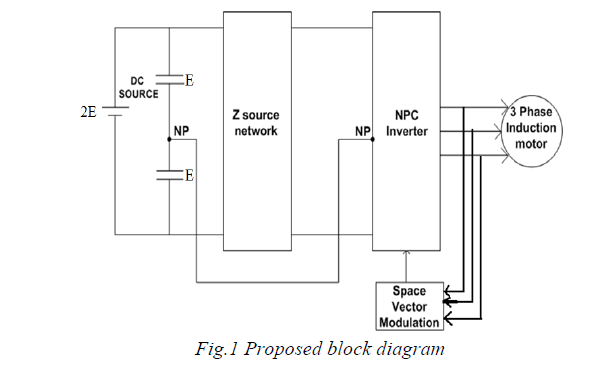A. Space Vector Pulse Width Modulation
At present, when fast and cheap semiconductor devices are available, it is more desirable to use the PWM technique in the low and middle power range since a PWM controlled inverter is able to control both the amplitude and frequency of the output voltage and does not require an additional voltage control on the DC side. This practice is based on multiple pulses in each half AC period, with variable width. Pulse width modulation is the process of modifying the width of the pulses in a pulse train in direct proportion to a small control signal. There are different types of pulse width modulations. Among them, the well-known are Space vector pulse width modulation (SVPWM).
Space Vector PWM (SVPWM) is a more sophisticated technique for generating a fundamental sine wave that provides a higher voltage to the motor and Lower THD when compared to sinusoidal PWM. The space vectors technique is nowadays commonly known as space vector modulation (SVM).The switching vectors and sectors is shown in the figure 2. The table 1 shows the switching sequence of vectors for inverters.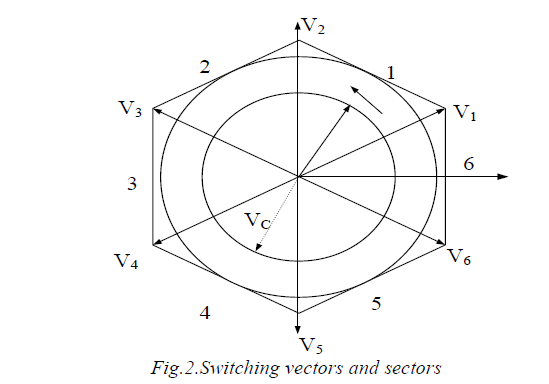The space vector pulse width modulation is used to generate the voltages applied to the stator phases. It uses a special scheme to switch the power transistors to generate pseudo sinusoidal currents in the stator phases. This method is increasingly used for AC drives with the condition that the harmonic current is as small as possible and the maximum output voltage is as large as possible.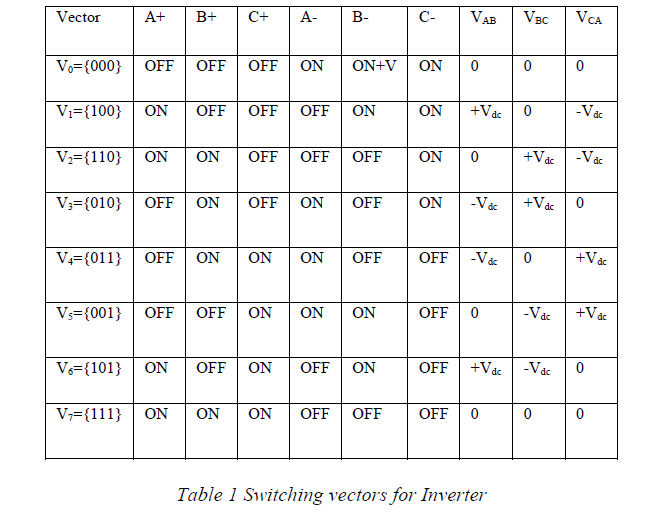B. Principle of Space Vector Modulation
Space vector modulation (SVM) is that which treats sinusoidal voltage as a rotating constant amplitude vector rotating with constant frequency. This PWM technique approximates the reference voltage Vref by a combination of the eight switching patterns. Coordinate Transformation (abc reference frame to the stationary α-β frame). That is a three-phase voltage vector is transformed into a vector in the stationary α-β coordinate frame represents the spatial vector sum of the three- phase voltage. The vectors (V1 to V6) divide the plane into six sectors which is generated by two adjacent non-zero vectors.
To implement the space vector PWM, the abc reference of the voltage equations can be transformed into the stationary αβ reference frame which consists of the horizontal (α) and vertical (β) axes, as a result, six non-zero vectors and two zero vectors are possible. Six nonzero vectors (V1 - V6) shape the axes of a hexagonal as depicted in figure 2 and feed electric power to the load or DC link voltage is supplied to the load. The angle between any adjacent two nonzero vectors is 60 degrees. Meanwhile, two zero vectors (V0 and V7) are at the origin and apply zero voltage to the load. The vectors are called the basic space vectors which are mentioned by V0, V1, V2, V3, V4, V5, V6, and V7.
The same transformation can be applied to the desired output voltage to get the desired reference voltage vector Vref in the d-q plane. The objective of space vector PWM technique is to approximate the reference voltage vector Vref using the eight switching patterns. The space vector pulse width modulation is used to generate the voltages applied to the stator phases. It uses a special scheme to switch the power transistors to generate pseudo sinusoidal currents in the stator phases. The space vectors technique is nowadays commonly known as space vector modulation (SVM).
The SVPWM switching pattern is shown in the figure 3. It consists of eight vectors starting from V0 to V7.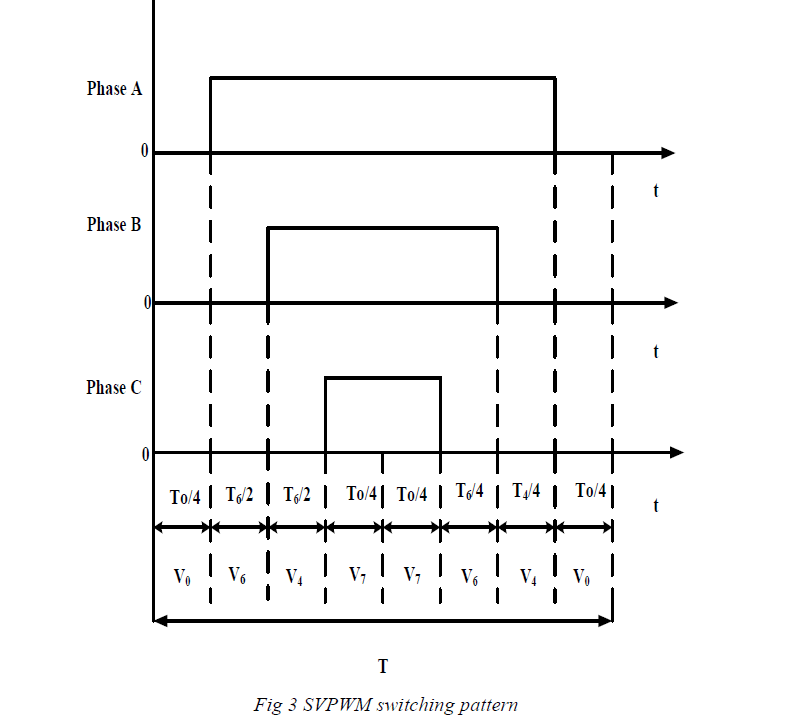### III.CIRCUIT ANALYSIS OF Z SOURCE NPC INVERTER

Among the three-level Z-source power converter topologies reported to date, the Z-source NPC inverter implemented using a single LC impedance network is considered to be an optimized topology in terms of component count. Referring to the REC Z-source NPC inverter is supplied with a split dc source. The middle point O is taken as a reference. When the REC Z-source NPC inverter is operated without any shoot-through states, then Vi is similar to 2E. As noted earlier, with this kind of operation, the maximum obtainable output line-to-line voltage cannot exceed the available dc source voltage (2E). Therefore, to obtain an output line-to-line voltage higher than 2E, shoot-through states are carefully inserted into selected phase legs to boost the input voltage to Vi>2E before it is inverted by the NPC circuitry. Thus, the REC Z-source inverter can boost and buck the output line-to-line voltage with a single-stage structure.
Two new switching states namely the UST and LST states were identified with the FST state and the nonshoot- through (NST) states (P, O, and N). Although operation using the FST and NST states is possible, it is generally preferable to use the UST and LST states in place of the FST states.The ULST operating mode is preferred because it produces an output voltage with enhanced waveform quality. The simplest FST operating mode requires all four switches in a phase leg to be turned ON. This is not minimal loss approach since, for example, switching phase A from +E through FST to 0 V would require switches {Qa1, Qa2, Qa’1, Qa’2} changing from {ON, ON, OFF, OFF} through {ON, ON, ON, ON} to {OFF, ON, ON, OFF}.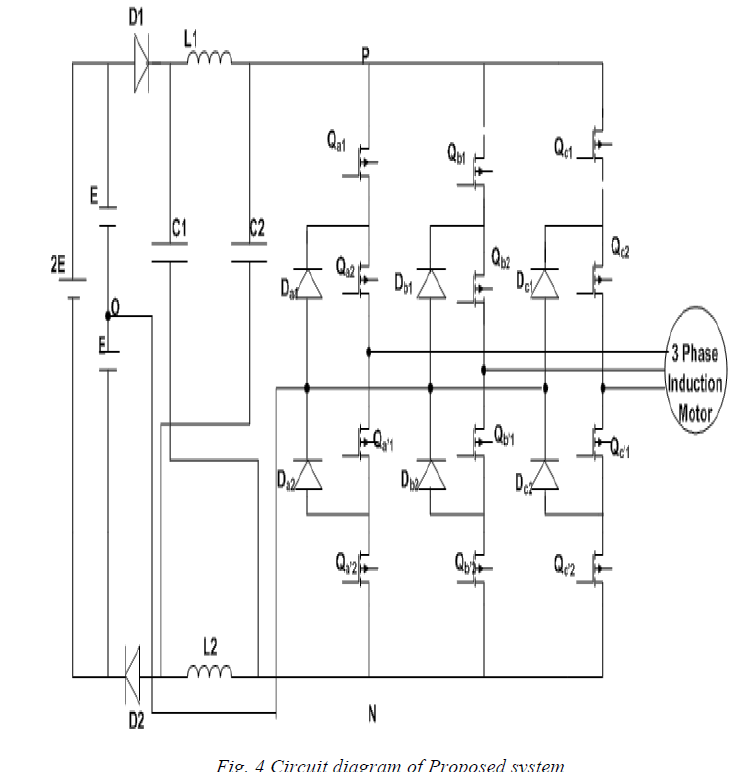An alternative FST operating mode which gives minimal loss uses two phase legs to create the shoot-through path. This requires, for example, synchronization of the turn ON instants of switches Qa1 from phase A and Qc’2 from phase C at the start of an FST state.Time interval during which switches {Qa1, Qa2, Qa’1} from phase A and {Qc2, Qc’1, Qc’2} from phase C are gated ON simultaneously to create a shoot-through path. However, the output line-to-line voltage obtained using the minimal loss FST approach has higher harmonic distortion (compared to the ULST approach) in its output voltage waveform because the voltage levels produced do not have adjacent level switching. Therefore, in this paper, the ULST operating mode is used for controlling the REC Z-source NPC inverter. Fig shows the simplified equivalent circuit for the NST state, shows the UST and LST states. Note that there are multiple ways of creating the UST and LST states using different phases.

### IV.SIMULATION OF PROPOSED SYSTEM

A. Implementation of proposed system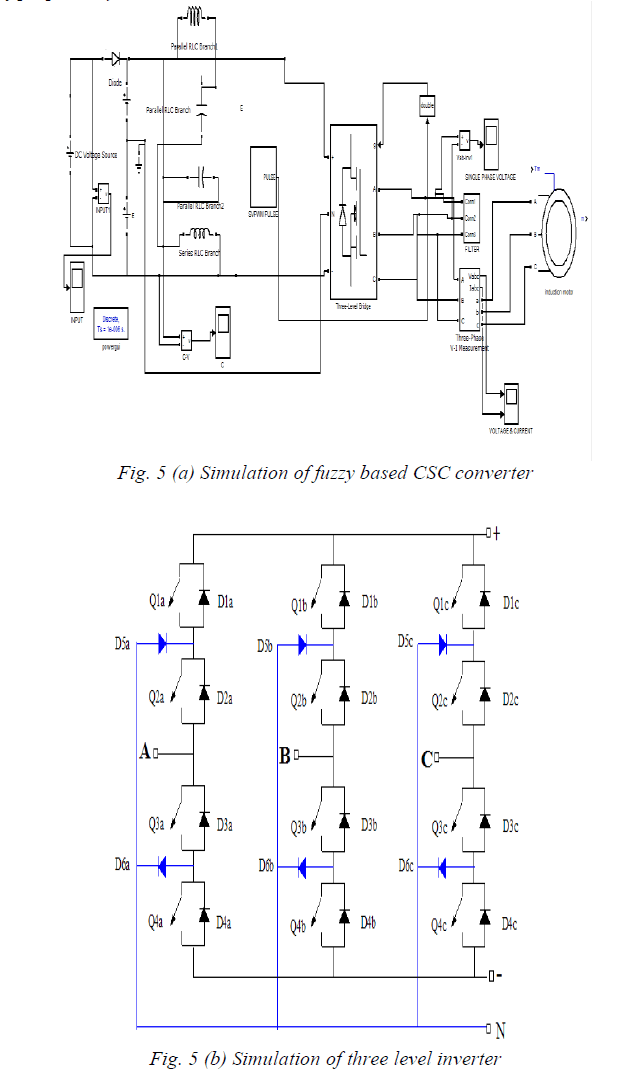### V.CONCLUSION

Modified Space Vector Modulation for a Z-source NPC inverter is presented. Using carefully inserted UST and LST states to the traditional NPC inverter state sequence, the Z-Source NPC inverter functions with the correct volt–second average and voltage boosting capability regardless of the angular position, by insertion of the shoot through states the number of device commutations was kept at a minimum sampling period, that needed by a traditional NPC inverter. The proposed concepts have been verified in simulations using a three-phase Z-source NPC inverter.

### REFERENCES

 S. Busquets-Monge, J. Rocabert, P. Rodriguez, S. Alepuz, and J. Bordonau, “Multilevel diode-clamped converter for photovoltaic generators with independent voltage control of each solar array,” IEEE Trans .Ind. Electron., vol. 55, no. 7, pp. 2713–2723, Jul. 2008.

 J. Zhao, Y. Han, X. He, C. Tan, J. Cheng, and R. Zhao, “Multilevel circuit topologies based on the switched-capacitor converter and diodeclamped converter,” IEEE Trans. Power Electron., vol. 26, no. 8, pp. 2127–2136,Aug. 2011.

 J.-S. Hu, K.-Y.Chen, T.-Y.Shen, and C.-H. Tang, “Analytical solutions of multilevel space-vector PWM for multiphase voltage source inverters, ”IEEE Trans. Power Electron., vol. 26, no. 5, pp. 1489–1502, May 2011.

 Z. Zhao, Y. Zhong, H. Gao, L. Yuan, and T. Lu, “Hybrid selective harmonic elimination PWM for common-mode voltage reduction in three level neutral-point-clamped inverters for variable speed induction drives, ”IEEE Trans. Power Electron., vol. 27, no. 3, pp. 1152–1158, Mar. 2012.

 P. Sun, C. Liu, J.-S. Lai, and C.-L. Chen, “Cascade dual buck inverter with phase-shift control,” IEEE Trans. Power Electron., vol. 27, no. 4,pp. 2067–2077, Apr. 2012.

 J. Ebrahimi, E. Babaei, and G.Gharehpetian, “A new topology of cascaded multilevel converters with reduced number of components for highvoltage applications,” IEEE Trans. Power Electron., vol. 26, no. 11, pp. 3109–3118, Nov. 2011.

 C. Govindaraju and K. Baskaran, “Efficient sequential switching hybrid modulation techniques for cascaded multilevel inverters,” IEEE Trans. Power Electron., vol. 26, no. 6, pp. 1639–1648, Jun. 2011.

 J. Shen, S. Schroeder, R. Roesner, and S. El-Barbari, “A comprehensive study of neutral-point self-balancing effect in neutral-point-clamped three level inverters,” IEEE Trans. Power Electron., vol. 26, no. 11, pp. 3084–3095, Nov. 2011.

 J. Li, J. Liu, D. Boroyevich, P. Mattavelli, and Y. Xue, “Three-level active neutral-point-clamped zero-current-transition converter for sustainable energy systems,” IEEE Trans. Power Electron., vol. 26, no. 12,pp. 3680–3693, Dec. 2011.

 H. Xiao and S. Xie, “Transformer less split-inductor neutral point clamped three-level PV grid-connected inverter,” IEEE Trans. Power Electron.,vol. 27, no. 4, pp. 1799–1808, Apr. 2012.